Solving Rational Equations MathBitsNotebook.com Terms of Use   Contact Person: Donna RobertsIn the Refresher section, we saw how to solve simple rational equations, and we touched on the concept of extraneous roots. We will now see more challenging problems and occurrences of extraneous roots.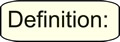A rational equation contains at least one rational expression.Remember, when solving rational equations, the trick is to clear the equation of denominators! Multiplying both sides by a common denominator will maintain a balanced equation, and get rid of those pesky denominators.Solving Rational Equations:  • Identify the least common denominator (LCD). • Multiply each side of the equation by the LCD. • Simplify (the denominators should be gone). • Solve the resulting equation. • Check the answers for possible extraneous roots.Example:
 1. Determine domain values that will create zero denominators. Given: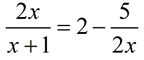Domain: x ≠-1, x ≠0 2. Find the least common denominator. In this example, the least common denominator is 2x(x + 1) or 2x2 + 2x. 3. Multiply both sides of the equation by the least common denominator. Be careful on the right hand side of the equation which contains subtraction. Be sure to distribute across the parentheses (across the subtraction). Reduce, simplify, and solve for x.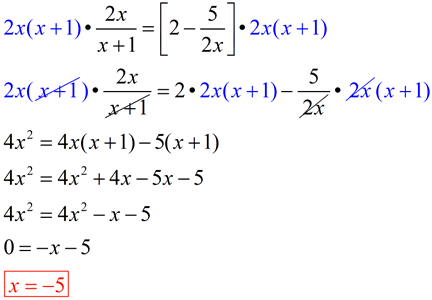4. Check to be sure that each answer does not cause a zero denominator problem in the original equation. From our domain check of the original equation (step 1), we know that the answers of x = -1 and 0 will NOT be solutions. Our solution should be OK. Show the check: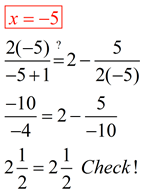Extraneous Roots:
 1. Determine domain values that will create zero denominators. Given: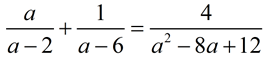Domain: a ≠2, a ≠6 2. Find the least common denominator. In this example, after factoring a2 - 8a + 12 into (a - 2)(a - 6), we can see that the least common denominator is a2 - 8a + 12 or (a - 2)(a - 6). 3. Multiply both sides of the equation by the least common denominator. Be careful on the left hand side of the equation which contains addition. Be sure to distribute across the parentheses (across the addition). Reduce, simplify, and solve for a.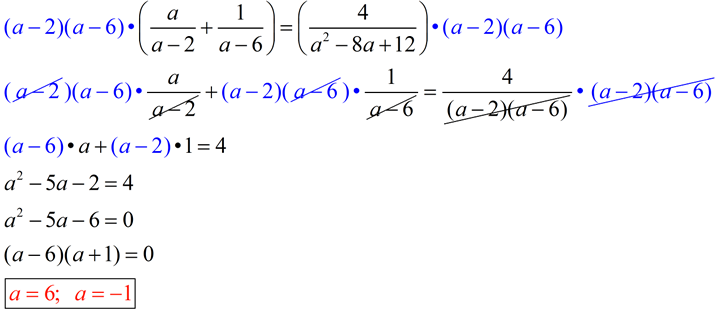4. Check to be sure that each answer does not cause a zero denominator problem in the original equation. From our domain check of the original equation (step 1), we know that the answer of a = 6 will NOT be a solution, since it causes a zero denominator problem. The only possible solution is a = -1. Show the checks: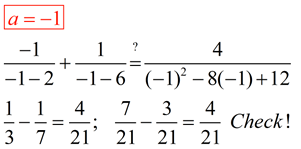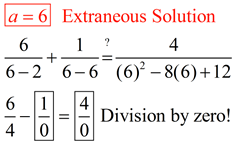5. Visual confirmation! Let's look at the graphs to see that the original equation is not quite the same as the manipulated equation (the derived equation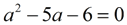).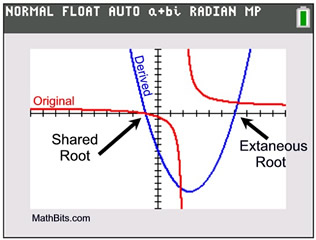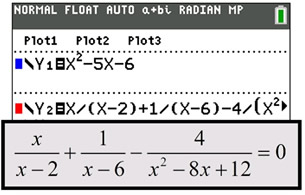Subtract the right side from the left side and graph to see the original equation.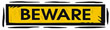Any time you multiply each side of an algebraic equation, you risk the introduction of an extraneous solution. This is a solution of the derived equation (the one you created by multiplying), but not a solution of the original equation. When working with rational equations you must check ALL solutions back into the original equation to determine if they are true solutions or extraneous solutions.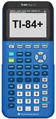Check out how to use your graphing calculator with the rational equations. Click here.